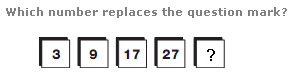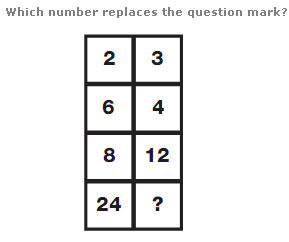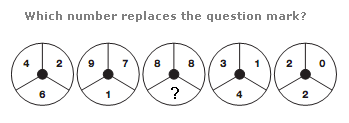# Puzzles - Number puzzles

### Exercise :: Number puzzlesAnswer : 39 Explanation : Moving from left to right, add 6 to the first number to get the next one along, then 8, 10 and 12 to complete the sequence.Answer : 16 Explanation : Starting with the top left number, and working down one row at a time, alternating between left and right, double the number each time. Repeat this sequence, starting with the top right number.Answer : 1 Explanation : Numbers in segments of the central circle equal the difference between the sum of the numbers in corresponding segments of the left two and right two circles.